# How to use The Flex Sensor

The flex sensor is a variable resistor that can bend. The resistance increases as you bend the component to a smaller angle. This component can be used to measure how much someone is bending their fingers or when a door is opened through this technology.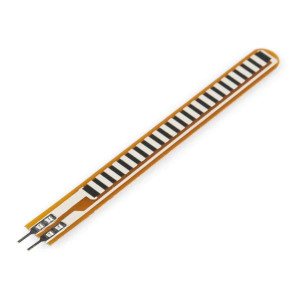## How it works​

One side of the sensor is created with polymer ink which is a conductive material. When the sensor is straight and not bent the resistance is around 30Ω. When you begin to bend the flex sensor away from the ink side the resistance increases to 50-70Ω.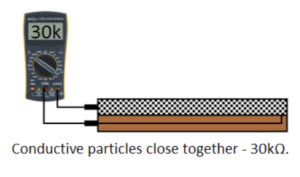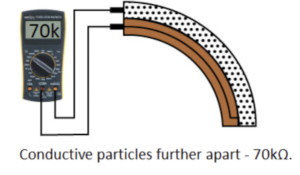## Wiring​

The sensor is not polarized meaning you can wire the component however you like.

• Pin 1: connected to Arduino pin A0 and is Grounded
• Pin 2: connected to voltage from the Arduino (5V)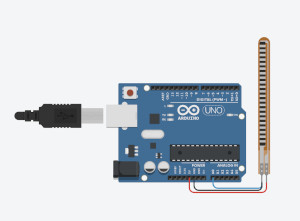## Wiring Guide​

• Connect the 5V and GND pins to the breadboard
• Connect a 10 kΩ resistor to one of the Flex sensor pins
• Connect the same Flex sensor pin to an Analog pin on the Arduino Uno
• Connect the other end of the resistor to ground
• Connect the other Flex sensor pin to voltage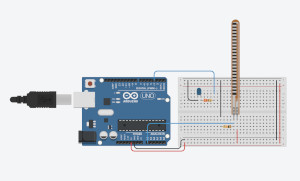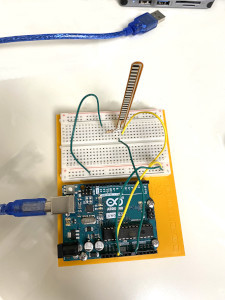## Programming​

The following code demonstrates how the Flex sensor works and measures the resistance when it is bent. If you want to skip the steps, you can jump to the Full Code.

Step 1: Create variables for the sensors pin and a variable to store the data that is collected

``int flex = A0;int data = 0;``

Step 2: Set up serial communication, set the flex pin as an OUTPUT.

``void setup() { Serial.begin(9600); pinMode(flex, INPUT);}``

Step 3: Read the data from the flex pin and print it out to the serial monitor

``void loop() { data = analogRead(flex); Serial.println(data); delay(100); // one second delay}``

## Full Code​

``int flex = A0;int data = 0;void setup() { Serial.begin(9600); pinMode(flex, INPUT);}void loop() { data = analogRead(flex); Serial.println(data); delay(100); // one second delay}``

## Output​

The following code will result with the servo motor moving the number of degrees that is measured from the rotation of the potentiometer.